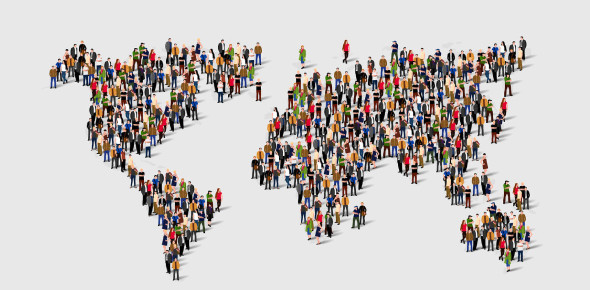# Population Density And Population Distribution Quiz

20 Questions | Total Attempts: 6669SettingsThis quiz tests your knowledge of population, population density, population distribution and population growth.

• 1.
The number of people living in a particular area at a particular point in time is known as the __________ of that area.
• A.

Population density

• B.

Population distribution

• C.

Population

• D.

Population growth

• 2.
This term refers to the way in which people are spread out over an area.
• A.

Population density

• B.

Population distribution

• C.

Population

• D.

Population growth

• 3.
The statement "250 per square kilometer" most likely refers to:
• A.

Population density

• B.

Population distribution

• C.

Population

• D.

Population growth

• 4.
Which of the following is NOT a factor affecting population distribution?
• A.

Relief

• B.

Fertility rate

• C.

Climate

• D.

Mineral deposits

• 5.
Antarctica is largely uninhabited. This is mainly because:
• A.

There is a lack of mineral resources

• B.

There is not much flat land

• C.

The soil is infertile

• D.

The climate is harsh

• 6.
The number of children a woman is expected to bear in her lifetime is known as the:
• A.

Birth rate

• B.

Fertility rate

• C.

Child expectancy

• D.

Population growth rate

• 7.
An increase in the birth rate will tend to cause the population to grow at a faster rate.
• A.

True

• B.

False

• 8.
An increase in the death rate will tend to slow down the rate of population growth.
• A.

True

• B.

False

• 9.
An increase in life expectancy from 65 to 75 will tend to slow down the rate of population growth.
• A.

True

• B.

False

• 10.
If immigration is far greater than emigration in a particular country, this will tend to cause the population of that country to grow.
• A.

True

• B.

False

• 11.
A drastic decrease in infant mortality will tend to cause the population to decrease.
• A.

True

• B.

False

• 12.
A population pyramid with a very wide base is a sign that the population is decreasing.
• A.

True

• B.

False

• 13.
Which of the following factors has the greatest impact on population distribution in Dominica?
• A.

Climate

• B.

Mineral deposits

• C.

Drainage and soils

• D.

Relief

• 14.
Most of Dominica's population lives:
• A.

In the interior

• B.

Near the coast

• C.

In forested areas

• D.

In the northern tip of the island

• 15.
The highest population densities in Dominica exist in and around:
• A.

Roseau

• B.

Marigot

• C.

Portsmouth

• D.

Salisbury

• 16.
In recent decades, the rate of Jamaica's population growth has been:
• A.

Increasing slowly

• B.

Decreasing

• C.

Increasing rapidly

• D.

Stable

• 17.
In recent decades, Jamaicas fertility rate and birth rate have decreased.
• A.

True

• B.

False

• 18.
In Jamaica, immigration is far greater than emigration.
• A.

True

• B.

False

• 19.
The population of the USA is growing very slowly.
• A.

True

• B.

False

• 20.
The main factor responsible for the growth of the US population is immigration.
• A.

True

• B.

False

Related TopicsBack to top